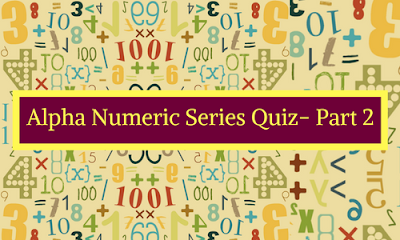# Alpha Numeric Series Quiz- Part 2Directions (Q.1-5): Study the following arrangement and answer the question given below:
U P @ 2 K A © M 1 H 3 D # 6 £ 8 S C 5 % 9 E 4 O \$ Q 7 I

### Question.1

If all the symbols are deleted from then what is the position of D?
A. Tenth from the left end
B. Thirteenth from the right end
C. Nineteenth from the left end
D. (A) and (B)
E. Seventh from the right end

Ans. D.
Solution:
U P 2 K A M 1 H 3 D 6 8 S C 5 9 E 4 O Q 7 I

### Question.2

Complete the series:
A. E%O
B. C89
C. 865
D. S£%
E. None of these

Ans. C.
Solution:
865

### Question.3

Four of the following five are alike in a certain way based on their position. Which is the one that does not belong to that group?
A. 9%E
B. 2@P
D. D3#
E. S8C

Ans. B.
Solution:
2@P

### Question.4

How many such consonant are there in the above arrangement, each of which is immediately followed by a symbol?
A. Two
B. More than three
C. One
D. Three
E. None

Ans. A.
Solution:
P@, D#

### Question.5

Which of the following is the sixteenth to the left of the fifth from the right end of the given arrangement?
A. 8
B. %
C. 6
D. @
E. M

Ans. E.
Solution:
M 1 H 3 D # 6 £ 8 S C 5 % 9 E 4 O \$ Q 7 I#### What's trending in BankExamsToday

Smart Prep Kit for Banking Exams by Ramandeep Singh - Download here